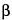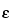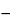Lutein

C40H56O2 568.87--Carotene-3,3¢-diol (3R,3¢R,6¢R)[127-40-2].
DEFINITION
Lutein is the purified fraction obtained from saponification of the oleoresin of Tagetes erecta L. It contains NLT 80.0% of total carotenoids calculated as lutein (C40H56O2). It contains NLT 74.0% of lutein and NMT 8.5% of zeaxanthin, both calculated as lutein (C40H56O2) on the anhydrous basis.
IDENTIFICATION
Wavelength range:  300–700 nm
Sample solution:   Prepare as directed for the Sample solution in the test for Content of Total Carotenoids.
Acceptance criteria:  It meets the requirements in the chapter. The absorbance ratio A446/A474 is 1.09–1.14.
•  B. The retention time for the major peak of the Sample solution corresponds to that of the Standard solution, as obtained in the test for Content of Lutein.
COMPOSITION
•  Content of Total Carotenoids
[Note—Use low-actinic glassware. ]
Diluent:  Hexanes, acetone, toluene, and dehydrated alcohol (10:7:7:6)
Sample stock solution:  0.3 mg/mL of Lutein in Diluent
Sample solution:  3.0 µg/mL of Lutein in dehydrated alcohol from dilution of Sample stock solution
Instrumental conditions
Mode:  UV-Vis
Analytical wavelength:  446 nm
Blank:  Dehydrated alcohol
Analysis
Sample:  Sample solution
Calculate the percentage of total carotenoids (T) as lutein (C40H56O2) in the portion of Lutein taken:
Result = A/(F × C)
 A = = absorbance of the Sample solution F = = coefficient of extinction (E1%) of lutein in alcohol (100 mL·g1·cm1), 2550 C = = concentration of lutein in the Sample solution (g/mL)
Acceptance criteria:   NLT 80.0%
•  Content of Lutein
Mobile phase:  Hexane and ethyl acetate (3:1)
Standard solution:  150 µg/mL of USP Lutein RS in Mobile phase
Sample solution:   Transfer 1 mL of the Sample stock solution from the test for Content of Total Carotenoids, and evaporate under a stream of nitrogen to dryness. Add 1 mL of Mobile phase, and sonicate to dissolve.
Chromatographic system
Mode:  LC
Detector:  UV-Vis at 446 nm
Column:  4.6-mm × 25-cm; 5-µm packing L3
Flow rate:  1.5 mL/min
Injection size:  10 µL
System suitability
Sample:  Standard solution
[Note—The relative retention times for lutein and zeaxanthin are about 1.0 and 1.05, respectively. ]
Suitability requirements
Resolution:  NLT 1.0 between lutein and zeaxanthin
Tailing factor:  NMT 2.0
Relative standard deviation:  NMT 2.0%
Analysis
Sample:  Sample solution
Calculate the percentage of the lutein peak as the total detected area in the portion of Lutein taken:
Result = (rU/rT) × 100
 rU = = peak response of lutein rT = = sum of the responses of all the peaks
Acceptance criteria:  NLT 85%
Calculate the percentage of lutein in the portion of Lutein taken:
Result = (rU/rT) × T
 rU = = individual peak response rT = = sum of the responses of all the peaks T = = percentage of total carotenoids as determined in the test for Content of Total Carotenoids
Acceptance criteria:  NLT 74.0% of lutein on the anhydrous basis
•  Zeaxanthin and Other Related Compounds
[Note—Use low-actinic glassware. ]
Mobile phase, Standard solution, Sample solution, and Chromatographic system:  Proceed as directed in Content of Lutein.
Analysis
Sample:  Sample solution
Calculate the percentage of zeaxanthin as the total detected area in the portion of Lutein taken:
Result = (rU/rT) × 100
 rU = = peak response of zeaxanthin rT = = sum of the responses of all the peaks
Acceptance criteria:  NMT 9.0%
Calculate the percentage of zeaxanthin in the portion of Lutein taken:
Result = (rU/rT) × T
 rU = = peak response of zeaxanthin rT = = sum of the responses of all the peaks T = = percentage of total carotenoids as determined in the test for Content of Total Carotenoids
Calculate the percentage of other related compounds in the portion of Lutein taken:
Result = (rU/rT) × 100
 rU = = individual peak response of any other peak in the chromatogram (excluding zeaxanthin and lutein) rT = = sum of the responses of all the peaks
Acceptance criteria:  NMT 8.5% of zeaxanthin; NMT 1.0% of any other single related compound on the anhydrous basis
IMPURITIES
SPECIFIC TESTS